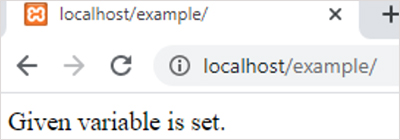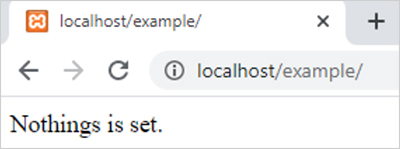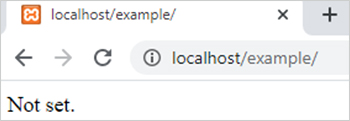isset function in PHP || PHP Functions# isset function in php

1983

Isset() function checks whether a variable is set or not. If the variable is set then it check the value of that variable and return TRUE if the value is not NULL.

Note
1. It is an inbuilt function of PHP.
2. The return type of this function is Boolean means it can return either TRUE or FALSE.
3. If we passed more than one variables, than this function return TRUE only if all variables are declared or set.

### isset() function Syntax:

`isset(variable1, .... , VariableN);`

Here variable1 to variableN are the variables which we can supplied to isset function.

Example:

```<?php
\$var ='set';
if (isset(\$var)) {
echo "Given variable is set.";  // Print this because var is set
}else{
echo "Nothing is set.";
}
?>
```

Output:Another Example:

```<?php
\$var=NULL;
if (isset(\$var)) {
echo "Given variable is set.";
}else{
echo "Nothing is set."; // Print this because var is set but the value is NULL
}
?>```

Output:Remarks: we can unset the value of a variable by using unset() function.

Another Example:

```<?php
\$var1="Coding Tag";
\$var2=NULL;
if (isset(\$var1,\$var2)) {
echo "Given variables are set.";
}else{
echo "Not set."; // Print this because var2 is NULL
}
?>```

Output:Share:## Big Hosting Days

Upto 75@ OFF on Web Hosting

## Get a .COM for just \$6.98

Secure Domain for a Mini Price